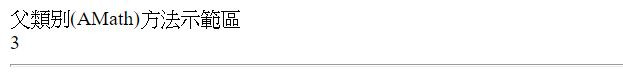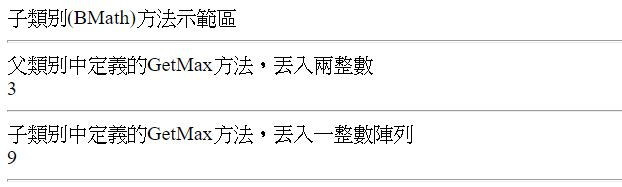#DAY 16
0
Modern Web

### ASP.NET Web Form 入門30天系列 第 16 篇

→「引數的型別、數目不同」，呼叫後「結果」是不同

``````<body>
<form id="form1" runat="server">
<div>
<asp:Label ID="message" runat="server" Text=""></asp:Label>
</div>
</form>
</body>
``````

``````class AMath
{
public int GetMax(int num1,int num2) //丟入的是兩個整數
{
if(num1>num2)
{return num1;}
else
{return num2;}
}
}
``````

``````message.Text += "父類別(AMath)方法示範區<br>";
AMath aMath = new AMath();
int a = 1;
int b = 3;
int aMathMax = aMath.GetMax(a, b);
message.Text += aMathMax + "<br><hr>";
````````````class BMath:AMath // BMath 繼承 AMath 類別
{
public int GetMax(int[] num) //丟入的是整數陣列
{
int max = num;
for(int i=1;i<num.Length;i++)
{
if(num[i]>max)
{max = num[i];}
}
return max;
}
}
``````

• 其繼承的 AMath 類別的 GetMax 方法
• 自己 BMath 類別修改的 GetMax 方法
``````message.Text += "子類別(BMath)方法示範區<br>";
BMath bMath = new BMath();
int a = 1;
int b = 3;
int[] c = {1,3,5,7,9};
int bMathMax_a = bMath.GetMax(a, b);
int bMathMax_b = bMath.GetMax(c);
message.Text += "父類別中定義的GetMax方法，丟入兩整數<br>";
message.Text += bMathMax_a + "<br><hr>";
message.Text += "子類別中定義的GetMax方法，丟入一整數陣列<br>";
message.Text += bMathMax_b + "<br><hr>";
``````